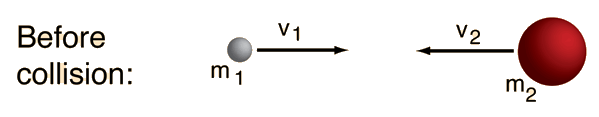# Collisions in One Dimension

In the general case of a one-dimensional collision between two masses, one cannot anticipate how much kinetic energy will be lost in the collision. Therefore, the velocities of the two masses after the collision are not completely determined by their velocities before the collision. However, conservation of momentum must be satisfied, so that if the velocity of one of the particles after the collision is specified, the other is determined.Initial conditions:

Mass m1 = kg , v1 = m/s.

Mass m2 = kg , v2 = m/s.

Initial momentum p = m1v1 + m2v2 = kg m/s .

Initial kinetic energy KE = 1/2 m1v12 + 1/2 m2v22 = joules.The following calculation expects you to enter a final velocity for mass m1 and then it calculates the final velocity of the other mass required to conserve momentum and calculates the kinetic energy either gained or lost to make possible such a collision. Keep in mind that the final velocities of the two masses cannot be predicted except in the ideal cases of perfectly elastic and perfectly inelastic collisions. If you do not enter a final velocity v'1, it will do a default routine to produce a number and then do the remainder of the calculation to be consistent with it. You can always change that default value and it will redo the calculation with the new value.

Final conditions:

 If the velocity of mass m1 is v'1 = m/s. You are expected to enter a chosen final value for the velocity of m1 here.

[Once v'1 is chosen, v'2 is determined by conservation of momentum.]

Then the velocity of mass m2 is v'2 = m/s

because the final momentum is constrained to be p' = m1v'1 + m2v'2 = kg m/s .

Final kinetic energy KE = 1/2 m1v'12 + 1/2 m2v'22 = joules.

For ordinary objects, the final kinetic energy will be less than the initial value. The only way you can get an increase in kinetic energy is if there is some kind of energy release triggered by the impact. Was one of your objects a hand grenade?

In the perfectly elastic case, the final velocities are constrained to be

 v'1(elastic) = m/s. v'2(elastic) = m/s You should be able to confirm from these velocity values that the velocity of separation after the collision is equal to the velocity of approach before the collision.

In the perfectly inelastic case where the two masses stick together, the final velocity of the combination will be

vinelastic = m/s

and the final kinetic energy will be

KEinelastic = joules.

A suggested exploration is to set the velocity of target mass m2 equal to zero and confirm that the fraction of kinetic energy retained in the perfectly inelastic case is equal to m1/(m1 + m2). This case is of practical value in the ballistic pendulum.

 Perfectly Inelastic Collisions

Calculation notes: This calculation is designed to calculate the velocity of the target particle after the collision, assuming that the projectile velocity after the collision is specified. Default values will be entered if no data entries are made, but mass and initial velocity values can be changed.

Index

Collision concepts

 HyperPhysics***** Mechanics R Nave
Go Back